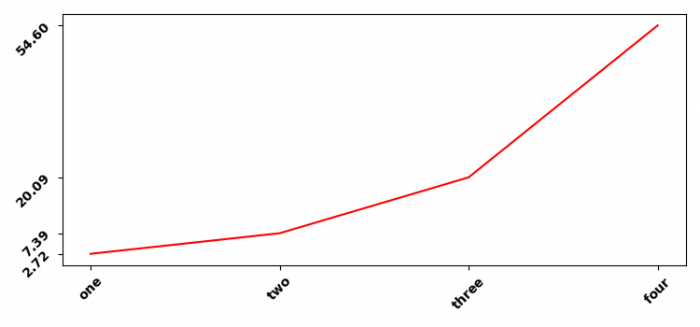# Bold font weight for LaTeX axes label in Matplotlib

To make bold font weight LaTeX axes label in matplotlib, we can take the following steps−

• Create x and y data points using numpy.
• Using subplot() method, add a subplot to the current figure.
• Set x and y ticks with data points x and y using set_xticks and set_yticks methods, respectively.
• Plot x and y using plot() method with color=red.
• To set bold font weight, we can use LaTeX representation.
• To display the figure, use show() method.

## Example

import numpy as np
from matplotlib import pyplot as plt, font_manager as fm
plt.rcParams["figure.figsize"] = [7.50, 3.50]
plt.rcParams["figure.autolayout"] = True
plt.rcParams["font.fantasy"] = "Comic Sans MS"
x = np.array([1, 2, 3, 4])
y = np.exp(x)
ax1 = plt.subplot()
ax1.set_xticks(x)
ax1.set_yticks(y)
ax1.plot(x, y, c="red")
ax1.set_xticklabels(["$\bf{one}$", "$\bf{two}$", "$\bf{three}$",
"$\bf{four}$"], rotation=45)
ax1.set_yticklabels(["$\bf{:.2f}$".format(y), "$\bf{:.2f}$".format(y),
"$\bf{:.2f}$".format(y), "$\bf{:.2f}$".format(y)], rotation=45)
plt.tight_layout()
plt.show()

## Output